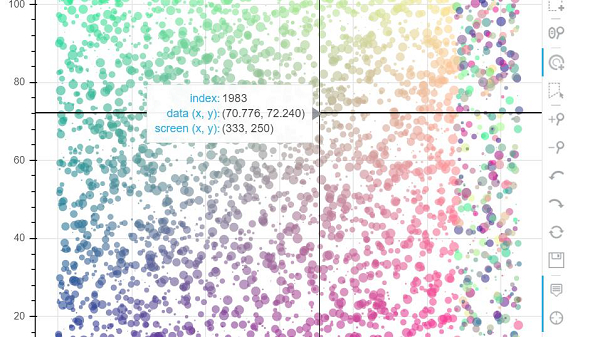# How can Bokeh be used to create a color scatter plot that shows data when hovering over points in Python?

PythonServer Side ProgrammingProgramming

Bokeh is a Python package that helps in data visualization. It is an open source project. Bokeh renders its plot using HTML and JavaScript. This indicates that it is useful while working with web−based dashboards.

Bokeh can be easily used in conjunction with NumPy, Pandas, and other Python packages. It can be used to produce interactive plots, dashboards, and so on.

Matplotlib and Seaborn produce static plots, whereas Bokeh produces interactive plots. This means when the user interacts with these plots, they change accordingly. Plots can be embedded as output of Flask or Django enabled web applications. Jupyter notebook can also be used to render these plots.

Dependencies of Bokeh −

Numpy
Pillow
Jinja2
Packaging
Pyyaml
Six
Python−dateutil

Installation of Bokeh on Windows command prompt

pip3 install bokeh

Installation of Bokeh on Anaconda prompt

conda install bokeh

Following is an example −

## Example

import numpy as np
from bokeh.plotting import figure, output_file, show

N = 5000
x = np.random.random(size=N) * 125
y = np.random.random(size=N) * 125
colors = [
"#%02x%02x%02x" % (int(r), int(g), 150) for r, g in zip(40+2*x, 30+2*y)
]

TOOLS="hover,crosshair,pan,wheel_zoom,zoom_in,zoom_out,box_zoom,undo,redo,reset,tap,save,box_select,poly_select,lasso_select,"
p = figure(tools=TOOLS)

fill_color=colors, fill_alpha=0.6,
line_color=None)

output_file("colorscatter.html", title="Color scatter plot")
show(p)

## Output## Explanation

• The required packages are imported, and aliased.

• The ‘random’ function is used to generate data.

• The figure function is called along with plot width and height.

• The ‘output_file’ function is called to mention the name of the html file that will be generated.

• The colors are defined as a list, to render colors to scatter plot data points.

• The ‘scatter’ function present in Bokeh is called, along with data.

• The ‘show’ function is used to display the plot.